# 「中高级前端」窥探数据结构的世界- ES6版## 1. 什么是数据结构？

### 1.1 为什么我们需要数据结构？

• 数据是计算机科学当中最关键的实体，而数据结构则可以将数据以某种组织形式存储，因此，数据结构的价值不言而喻。
• 无论你以何种方式解决何种问题，你都需要处理数据——无论是涉及员工薪水、股票价格、购物清单，还是只是简单的电话簿问题。
• 数据需要根据不同的场景，按照特定的格式进行存储。有很多数据结构能够满足以不同格式存储数据的需求。

### 1.2 八大常见的数据结构

1. 数组：`Array`
2. 堆栈：`Stack`
3. 队列：`Queue`
4. 链表：`Linked Lists`
5. 树：`Trees`
6. 图：`Graphs`
7. 字典树：`Trie`
8. 散列表（哈希表）：`Hash Tables`

• 堆栈和队列是类似于数组的结构，仅在项目的插入和删除方式上有所不同。
• 链表，树，和图 结构的节点是引用到其他节点。
• 散列表依赖于散列函数来保存和定位数据。

• 堆栈和队列是最简单的，并且可以从中构建链表。
• 树和图 是最复杂的，因为它们扩展了链表的概念。
• 散列表和字典树 需要利用这些数据结构来可靠地执行。

• 链表是记录和存储数据的最佳选择
• 而哈希表和字典树 在搜索和检索数据方面效果最佳。

## 2. 数组 - 知识补充

• 10,000个随机密钥在10,000个对象的数组中查找的执行效率对比图：
``````[
{
id: "key0",
content: "I ate pizza 0 times"
},
{
id: "key1",
content: "I ate pizza 1 times"
},
{
id: "key2",
content: "I ate pizza 2 times"
},
...
]

["key284", "key958", "key23", "key625", "key83", "key9", ... ]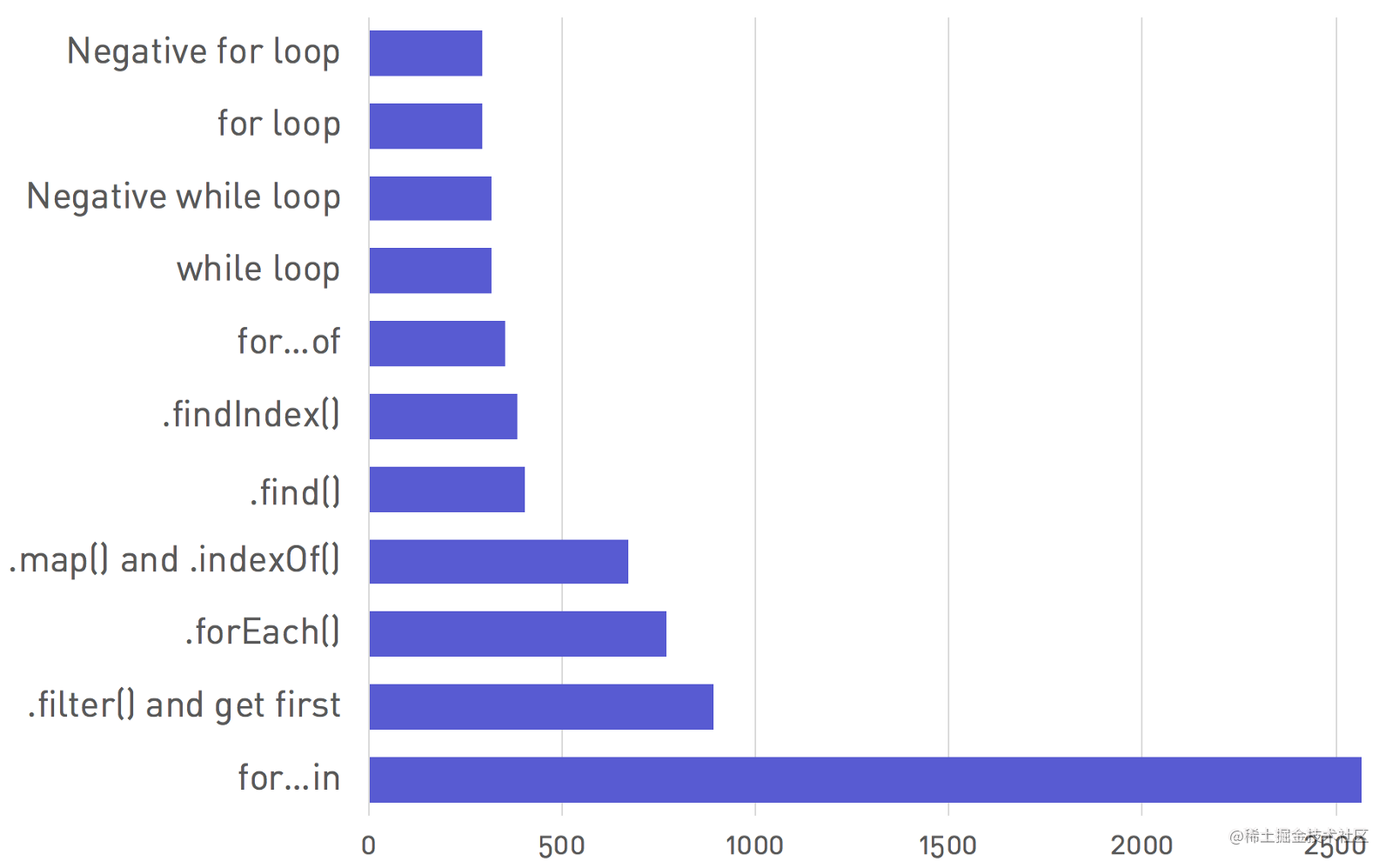### 2.1 `for... in`为何这么慢？

`for... in`语法令人难以置信的缓慢。在测试中就已经比正常情况下慢近9倍的循环。

## 3. 堆栈：`Stack`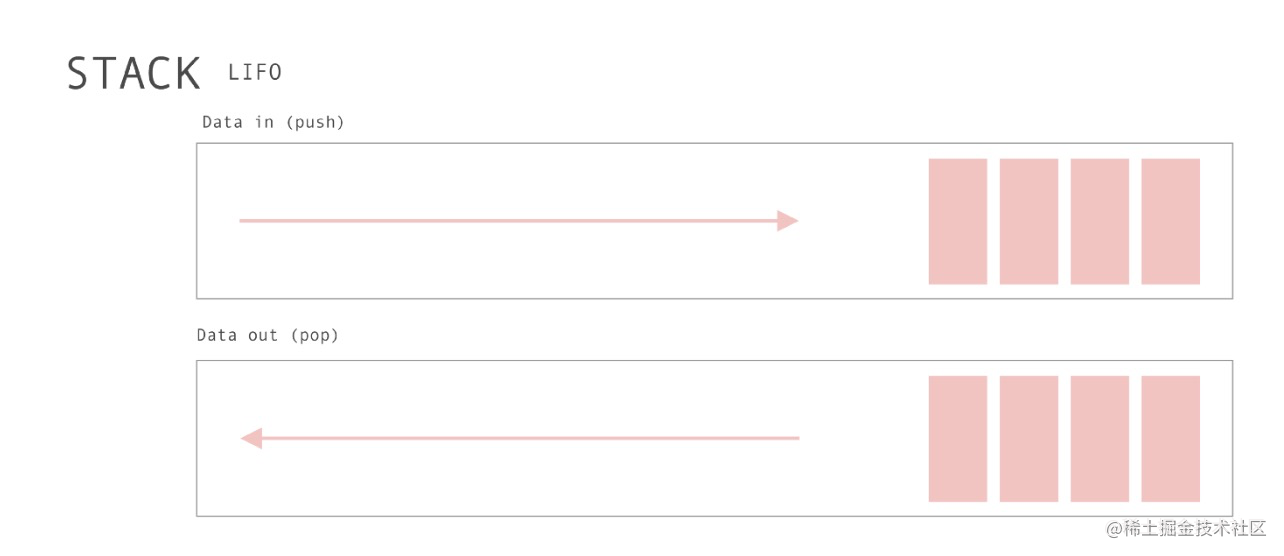堆栈是元素的集合，可以在顶部添加项目，我们有几个实际的堆栈示例：

• 浏览器历史记录
• 撤消操作
• 递归以及其它。

1. 两个原则操作：`push``pop``Push` 将元素添加到数组的顶部，而`Pop`将它们从同一位置删除。
2. 遵循"`Last In，First Out`"，即：`LIFO`，后进先出。
3. 没了。

### 3.1 堆栈的实现。

``````class Stack {
constructor(...items) {
this.reverse = false;
this.stack = [...items];
}

push(...items) {
return this.reverse
? this.stack.unshift(...items)
: this.stack.push(...items);
}

pop() {
return this.reverse ? this.stack.shift() : this.stack.pop();
}
}

const stack = new Stack(4, 5);
stack.reverse = true;
console.log(stack.push(1, 2, 3) === 5) // true
console.log(stack.stack ===[1, 2, 3, 4, 5]) // true

## 4. 队列：`Queue`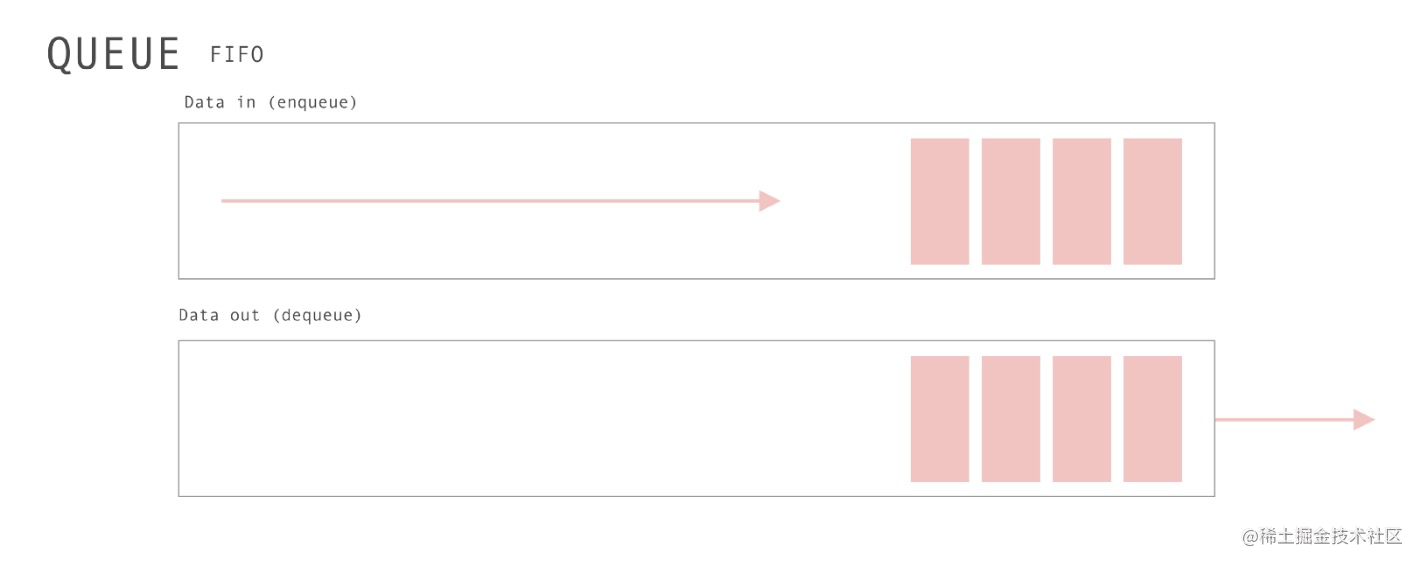而在前端开发中，最著名的队列使用当属浏览器/NodeJs中 关于宏任务与微任务，任务队列的知识。这里就不再赘述了。

• 只是具有两个主要操作的数组：`unshift``pop`
• 遵循`"Fist In，first out"`即：`FIFO`，先进先出。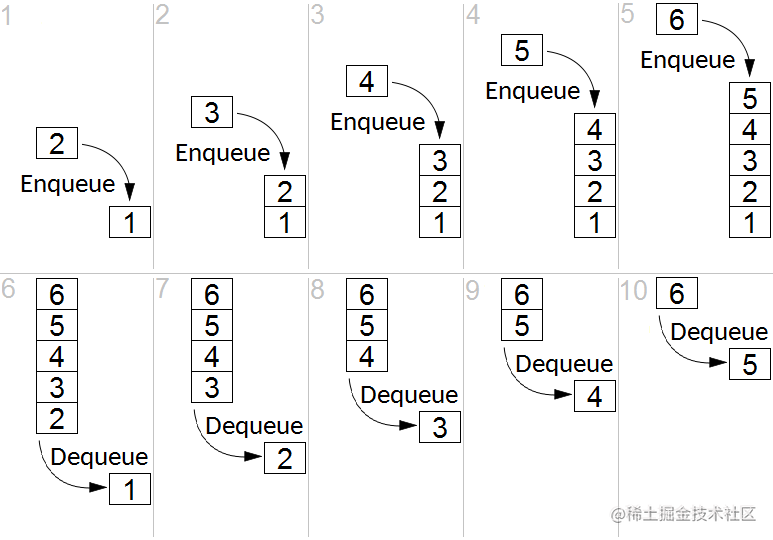### 4.1 队列的实现

``````class Queue {
constructor(...items) {
this.reverse = false;
this.queue = [...items];
}

enqueue(...items) {
return this.reverse
? this.queue.push(...items)
: this.queue.unshift(...items);
}

dequeue() {
return this.reverse ? this.queue.shift() : this.queue.pop();
}
}

## 5. 链表：`Linked Lists`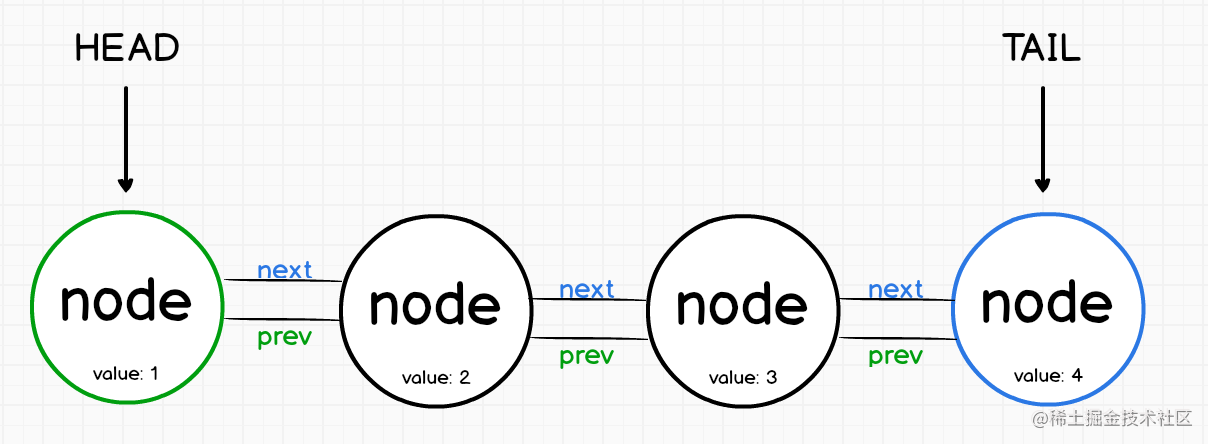第一个节点称为头部(`head`)，而最后一个节点称为尾部(`tail`)。

• 单链表是表示一系列节点的数据结构，其中每个节点指向列表中的下一个节点。
• 链表通常需要遍历整个操作列表，因此性能较差。
• 提高链表性能的一种方法是在每个节点上添加指向列表中上一个节点的第二个指针。
• 双向链表具有指向其前后元素的节点。

• 链接具有常量时间 插入和删除，因为我们可以只更改指针。
• 与数组一样，链表可以作为堆栈运行。

• 在客户端上，像`Redux`就以链表方式构建其中的逻辑。
• `React` 核心算法 `React Fiber`的实现就是链表。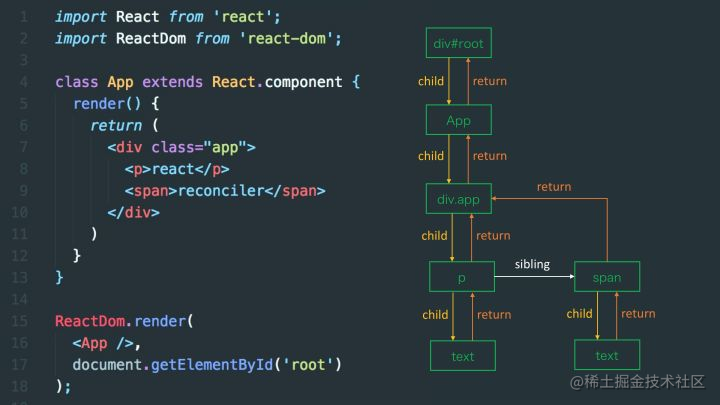• `React Fiber`之前的`Stack Reconciler`，是自顶向下的递归`mount/update`，无法中断(持续占用主线程)，这样主线程上的布局、动画等周期性任务以及交互响应就无法立即得到处理，影响体验。
• `React Fiber`解决过去`Reconciler`存在的问题的思路是把渲染/更新过程(递归diff)拆分成一系列小任务，每次检查树上的一小部分，做完看是否还有时间继续下一个任务，有的话继续，没有的话把自己挂起，主线程不忙的时候再继续。
• 在服务器上，像`Express`这样的`Web`框架也以类似的方式构建其中间件逻辑。当请求被接收时，它从一个中间件管道输送到下一个，直到响应被发出。

### 5.1 单链表实现

• `push（value）` - 在链表的末尾/头部添加一个节点
• `pop（）` - 从链表的末尾/头部删除一个节点
• `get（index）` - 返回指定索引处的节点
• `delete（index）` - 删除指定索引处的节点
• `isEmpty（）` - 根据列表长度返回true或false
• `print（）` - 返回链表的可见表示
``````class Node {
constructor(data) {
this.data = data
this.next = null
}
}

constructor() {
this.tail = null
// 长度非必要
this.length = 0
}
push(data) {
// 创建一个新节点
const node = new Node(data)
// 检查头部是否为空
if (this.head === null) {
this.tail = node
}
this.tail.next = node
this.tail = node
this.length++
}
pop(){
// 先检查链表是否为空
if(this.isEmpty()) {
return null
}
// 如果长度为1
if (this.head === this.tail) {
this.tail = null
this.length--
return this.tail
}
let node = this.tail
let currentNode = this.head
let penultimate

while (currentNode) {
if (currentNode.next === this.tail) {
penultimate = currentNode
break
}
currentNode = currentNode.next
}

penultimate.next = null
this.tail = penultimate
this.length --
return node
}

get(index){
// 处理边界条件
if (index === 0) {
}
if (index < 0 || index > this.length) {
return null
}

let currentNode = this.head
let i = 0
while(i < index) {
i++
currentNode = currentNode.next
}
return currentNode

}
delete(index){
let currentNode = this.head

if (index === 0) {
let deletedNode
deletedNode = currentNode
this.length--

return deletedNode
}

if (index < 0 || index > this.length) {
return null
}

let i = 0
let previous

while(i < index) {
i++
previous = currentNode
currentNode = currentNode.next
}
previous.next = currentNode.next
this.length--
return currentNode
}

isEmpty() {
return this.length === 0
}
print() {
const list = []
let currentNode = this.head
while(currentNode){
list.push(currentNode.data)
currentNode = currentNode.next
}
return list.join(' => ')
}
}

``````const l = new LinkedList()

// 添加节点
const values = ['A', 'B', 'C']
values.forEach(value => l.push(value))

console.log(l)
console.log(l.pop())
console.log(l.get(1))
console.log(l.isEmpty())
console.log(l.print())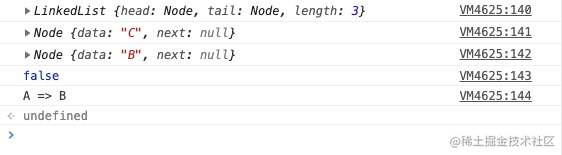### 5.2 双向链表实现

#### 1. 双向链表的设计

``````class Node {
constructor(data) {
// data 包含链表项应存储的值
this.data = data;
// next 是指向列表中下一项的指针
this.next = null;
// prev 是指向列表中上一项的指针
this.prev = null;
}
}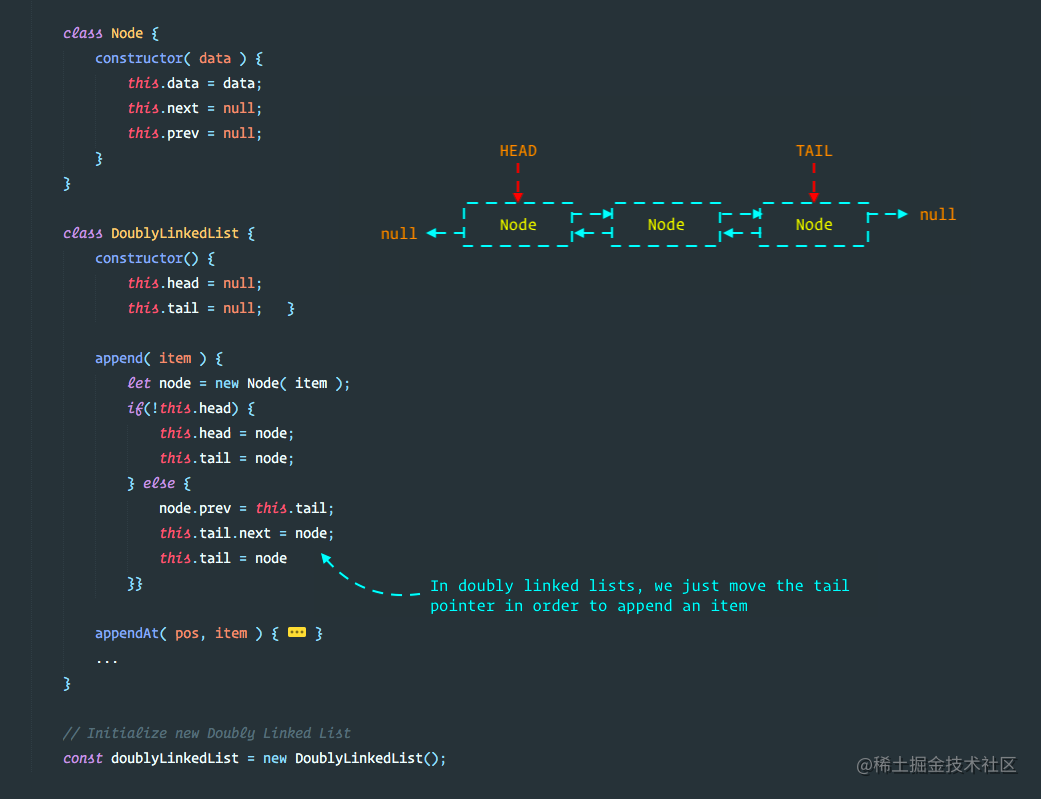还是敲一遍吧：

``````class DoublyLinkedList {
constructor() {
this.tail = null;
}
// 各种操作方法
// ...
}

#### 2. 双向链表的操作方法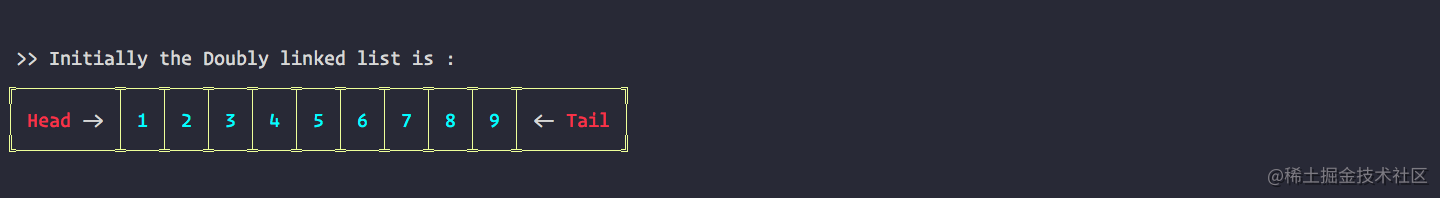• `Append & AppendAt`: 在链表的尾部/ 指定位置添加节点
``````append( item ) {
let node = new Node( item );
this.tail = node;
} else {
node.prev = this.tail;
this.tail.next = node;
this.tail = node
}
}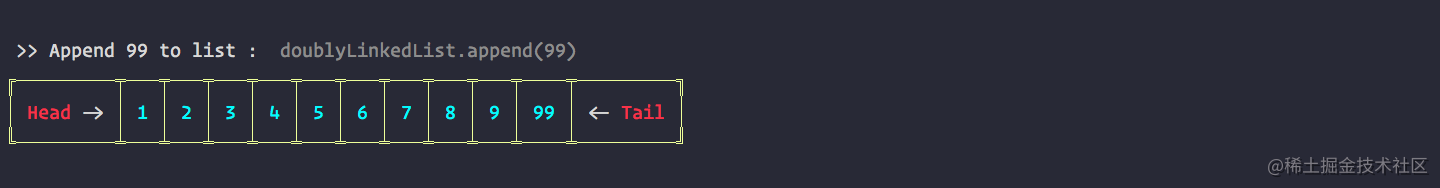``````appendAt( pos, item ) {
let current = this.head;
let counter = 1;
let node = new Node( item );
if( pos == 0 ) {
} else {
while(current) {
current = current.next;
if( counter == pos ) {
node.prev = current.prev
current.prev.next = node
node.next = current
current.prev = node
}
counter++
}
}
}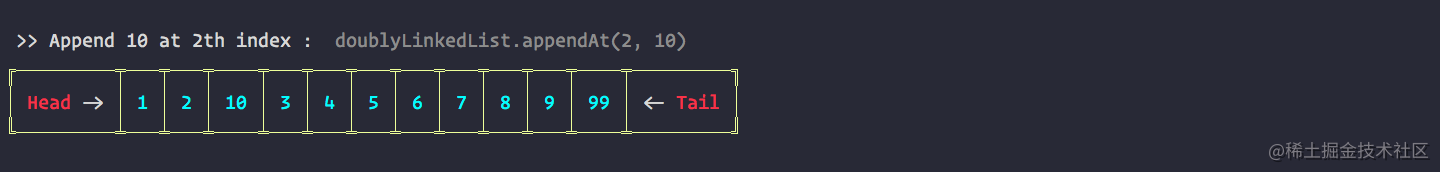• `Remove & RemoveAt`: 在链表的尾部/ 指定位置删除节点
``````remove( item ) {
let current = this.head;
while( current ) {
if( current.data === item ) {
if( current == this.head && current == this.tail ) {
this.tail = null;
} else if ( current == this.head ) {
} else if ( current == this.tail ) {
this.tail = this.tail.prev;
this.tail.next = null;
} else {
current.prev.next = current.next;
current.next.prev = current.prev;
}
}
current = current.next
}
}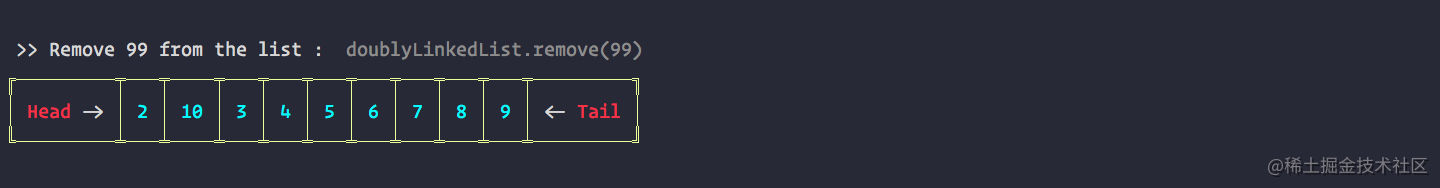``````removeAt( pos ) {
let current = this.head;
let counter = 1;
if( pos == 0 ) {
} else {
while( current ) {
current = current.next
if ( current == this.tail ) {
this.tail = this.tail.prev;
this.tail.next = null;
} else if( counter == pos ) {
current.prev.next = current.next;
current.next.prev = current.prev;
break;
}
counter++;
}
}
}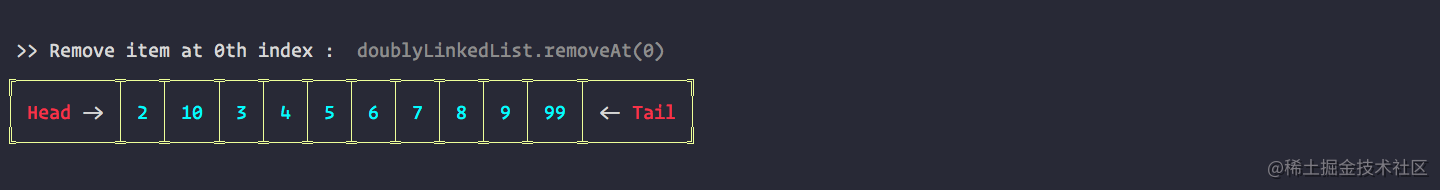• `Reverse`: 翻转双向链表
``````reverse(){
let current = this.head;
let prev = null;
while( current ){
let next = current.next
current.next = prev
current.prev = next
prev = current
current = next
}
}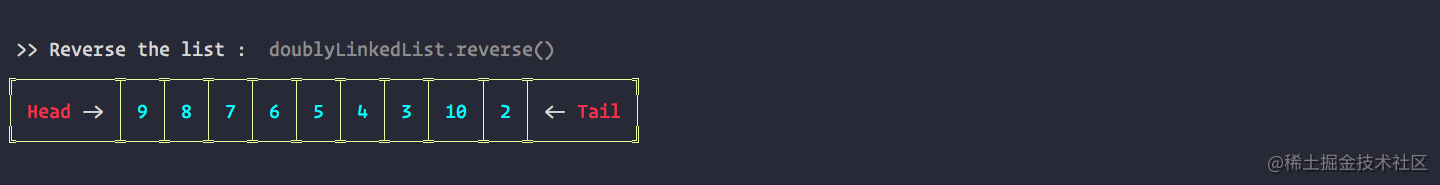• `Swap`：两节点间交换。
``````swap( nodeOne, nodeTwo ) {
let current = this.head;
let counter = 0;
let firstNode;
while( current !== null ) {
if( counter == nodeOne ){
firstNode = current;
} else if( counter == nodeTwo ) {
let temp = current.data;
current.data = firstNode.data;
firstNode.data = temp;
}
current = current.next;
counter++;
}
return true
}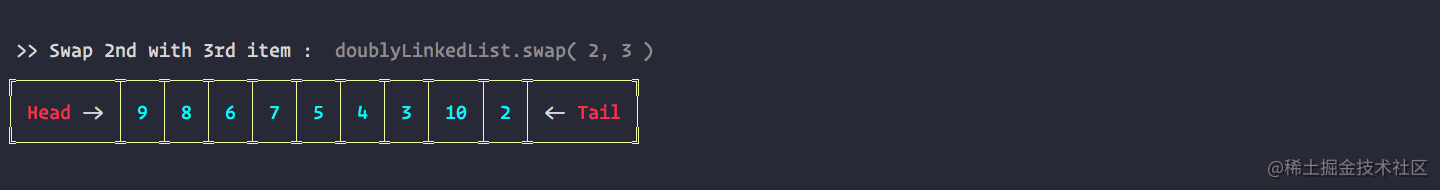• `IsEmpty & Length`：查询是否为空或长度。
``````length() {
let current = this.head;
let counter = 0;
while( current !== null ) {
counter++
current = current.next
}
return counter;
}
isEmpty() {
return this.length() < 1
}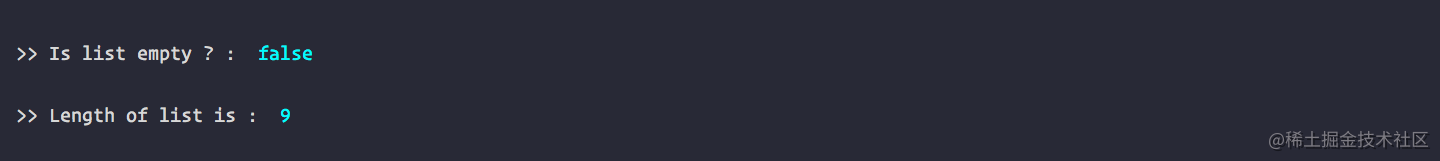• `Traverse`: 遍历链表
``````traverse( fn ) {
let current = this.head;
while( current !== null ) {
fn(current)
current = current.next;
}
return true;
}• `Search`：查找节点的索引。
``````search( item ) {
let current = this.head;
let counter = 0;
while( current ) {
if( current.data == item ) {
return counter
}
current = current.next
counter++
}
return false;
}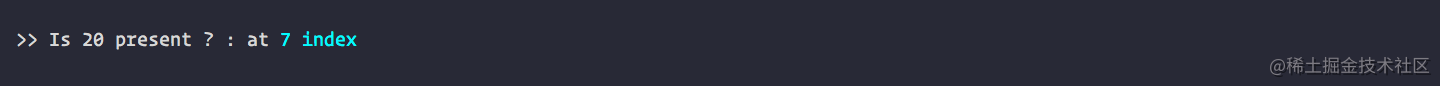## 6. 树：`Tree`

• 在树结构中，每一个结点只有一个父结点，若一个结点无父节点，则称为树的根结点，简称树的根（root）。
• 每一个结点可以有多个子结点。
• 没有子结点的结点称为叶子结点。
• 一个结点所拥有的子结点的个数称为该结点的度。
• 所有结点中最大的度称为树的度。树的最大层次称为树的深度。

### 6.1 树的分类

• 二叉树：`Binary Search Tree`
• AVL树：`AVL Tree`
• 红黑树：`Red-Black Tree`
• 线段树： `Segment Tree` - with min/max/sum range queries examples
• 芬威克树：`Fenwick Tree` (`Binary Indexed Tree`)

### 6.2 树的应用

1. `DOM`树。每个网页都有一个树数据结构。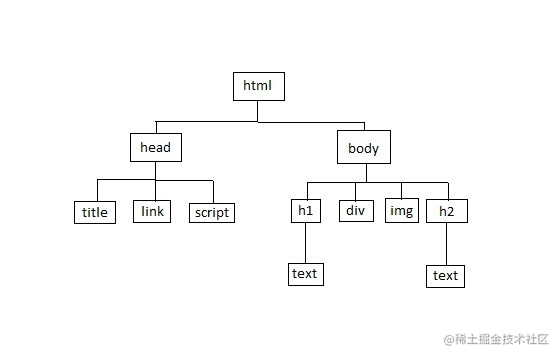2. `Vue``React``Virtual DOM`也是树。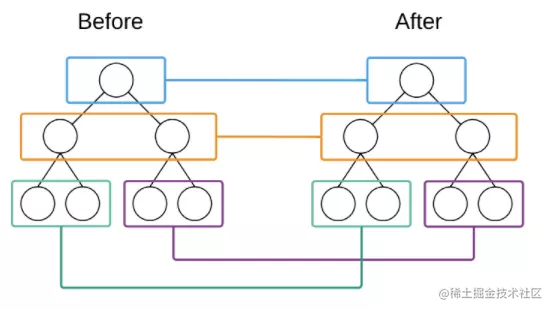### 6.3 二叉树：`Binary Search Tree`

• 二叉树是一种特殊的树，它的子节点个数不超过两个。
• 且分别称为该结点的左子树（left subtree）与右子树（right subtree）。
• 二叉树常被用作二叉查找树和二叉搜索树、或是二叉排序树（BST）。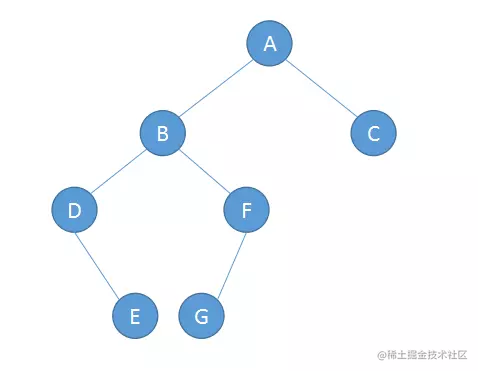### 6.4 二叉树的遍历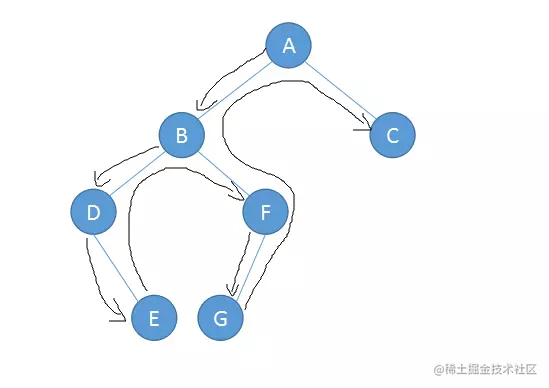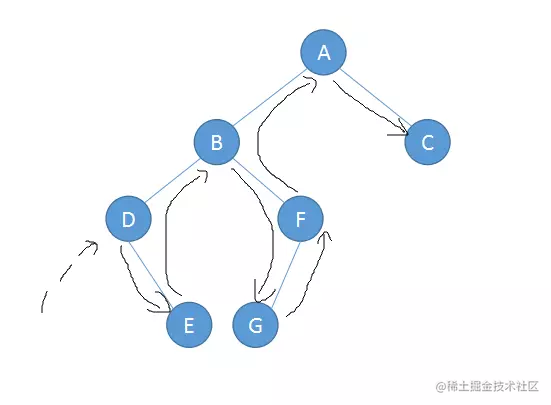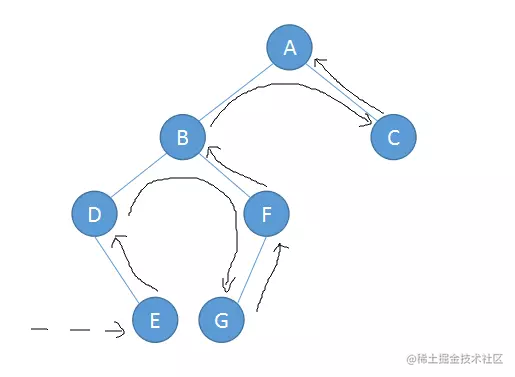• 前序遍历：ABDEFGC
• 中序遍历：DEBGFAC
• 后序遍历：EDGFBCA

### 6.5 二叉树的实现

``````class Node {
constructor(data) {
this.left = null
this.right = null
this.value = data
}
}

class BST {
constructor() {
this.root = null
}
// 二叉树的各种操作
// insert(value) {...}
// insertNode(root, newNode) {...}
// ...

#### 1. `insertNode`& `insert`：插入新子节点/节点

``````  insertNode(root, newNode) {
if (newNode.value < root.value) {
// 先执行无左节点操作
(!root.left) ? root.left = newNode : this.insertNode(root.left, newNode)
} else {
(!root.right) ? root.right = newNode : this.insertNode(root.right, newNode)
}
}

insert(value) {
let newNode = new Node(value)
// 如果没有根节点
if (!this.root) {
this.root = newNode
} else {
this.insertNode(this.root, newNode)
}
}

#### 2. `removeNode`& `remove`：移除子节点/节点

``````  removeNode(root, value) {
if (!root) {
return null
}

// 从该值小于根节点开始判断
if (value < root.value) {
root.left = this.removeNode(root.left, value)
return root
} else if (value > root.value) {
root.right = tis.removeNode(root.right, value)
return root
} else {
// 如果没有左右节点
if (!root.left && !root.right) {
root = null
return root
}

// 存在左节点
if (root.left) {
root = root.left
return root
// 存在右节点
} else if (root.right) {
root = root.right
return root
}

// 获取正确子节点的最小值以确保我们有有效的替换
let minRight = this.findMinNode(root.right)
root.value = minRight.value
// 确保删除已替换的节点
root.right = this.removeNode(root.right, minRight.value)
return root
}
}

remove(value) {
if (!this.root) {
return 'Tree is empty!'
} else {
this.removeNode(this.root, value)
}
}

#### 3. `findMinNode`:获取子节点的最小值

``````findMinNode(root) {
if (!root.left) {
return root
} else {
return this.findMinNode(root.left)
}
}

#### 4. `searchNode` & `search`：查找子节点/节点

``````searchNode(root, value) {
if (!root) {
return null
}

if (value < root.value) {
return this.searchNode(root.left, value)
} else if (value > root.value) {
return this.searchNode(root.right, value)
}

return root
}

search(value) {
if (!this.root) {
return 'Tree is empty'
} else {
return Boolean(this.searchNode(this.root, value))
}
}

1. `Pre-Order`：前序遍历
``````preOrder(root) {
if (!root) {
return 'Tree is empty'
} else {
console.log(root.value)
this.preOrder(root.left)
this.preOrder(root.right)
}
}

1. `In-Order`：中序遍历
``````inOrder(root) {
if (!root) {
return 'Tree is empty'
} else {
this.inOrder(root.left)
console.log(root.value)
this.inOrder(root.right)
}
}

1. `Post-Order`：后序遍历
``````postOrder(root) {
if (!root) {
return 'Tree is empty'
} else {
this.postOrder(root.left)
this.postOrder(root.right)
console.log(root.value)
}
}

## 7. 图：`Graph`

Graphs—-A Visual Introduction for Beginners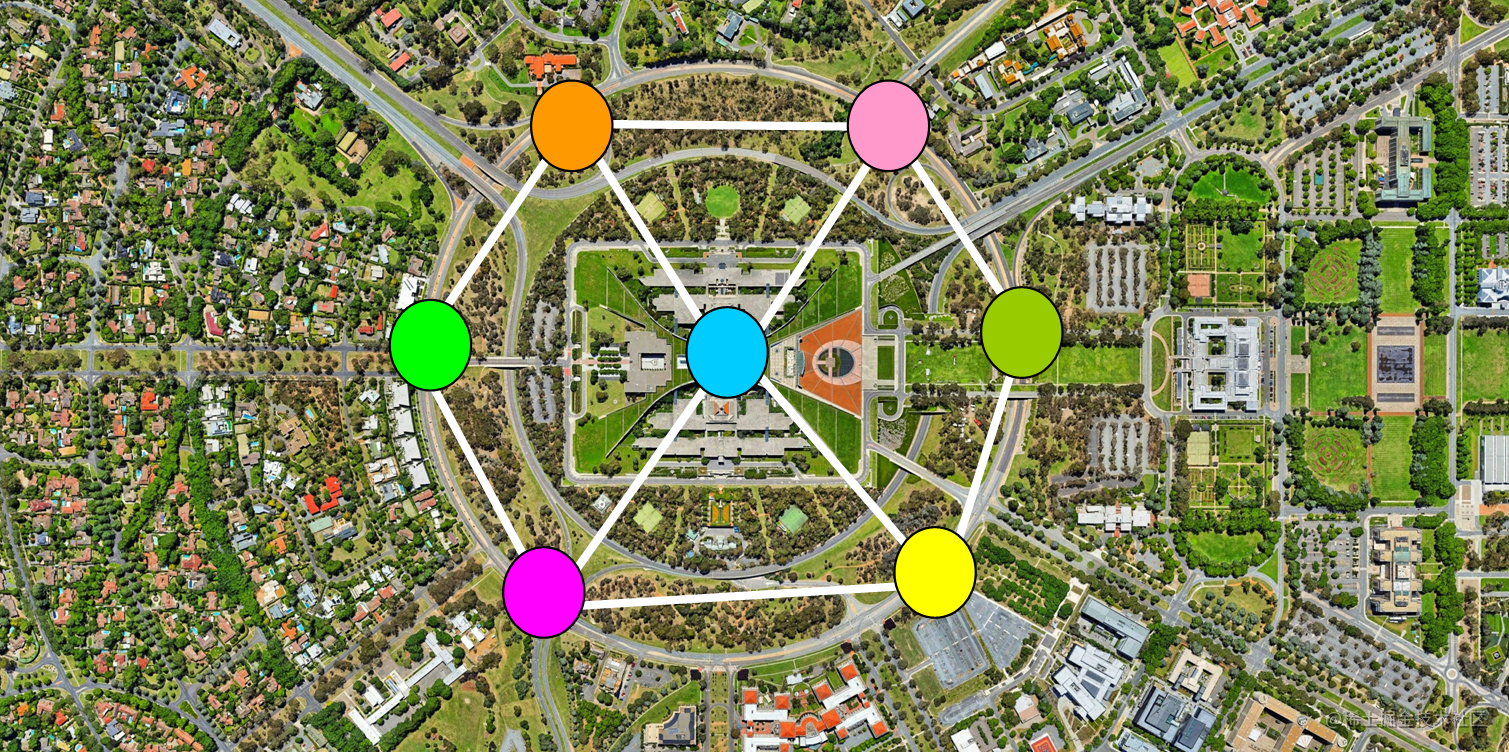### 7.1 图的应用

• 使用搜索服务，如`Google`，百度。
• 使用`LBS`地图服务，如高德，谷歌地图。
• 使用社交媒体网站，如微博，`Facebook`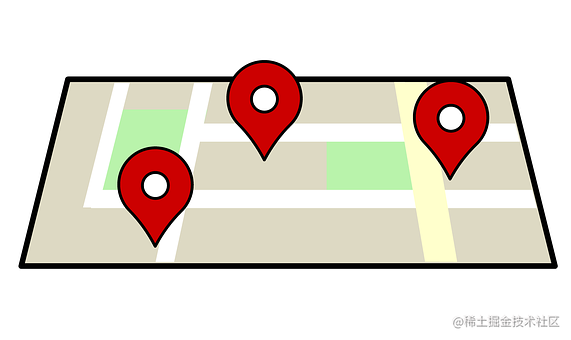• `GPS`系统和谷歌地图使用图表来查找从一个目的地到另一个目的地的最短路径。
• 社交网络使用图表来表示用户之间的连接。
• `Google`搜索算法使用图 来确定搜索结果的相关性。
• 运营研究是一个使用图 来寻找降低运输和交付货物和服务成本的最佳途径的领域。
• 甚至化学使用图 来表示分子！

### 7.2 图的构成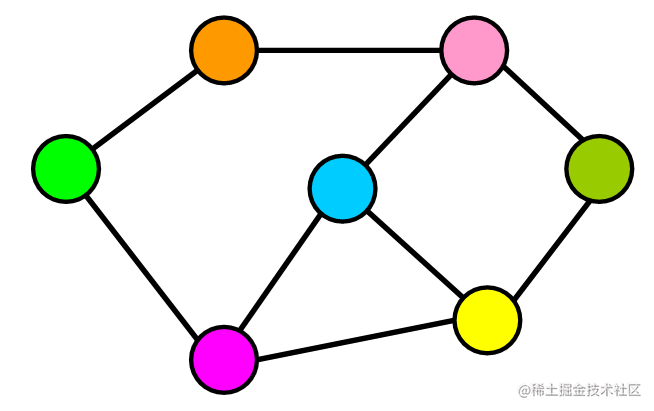#### 1. 图的基本元素

• 节点：`Node`，比如地铁站中某个站/多个村庄中的某个村庄/互联网中的某台主机/人际关系中的人.
• 边：`Edge`，比如地铁站中两个站点之间的直接连线, 就是一个边。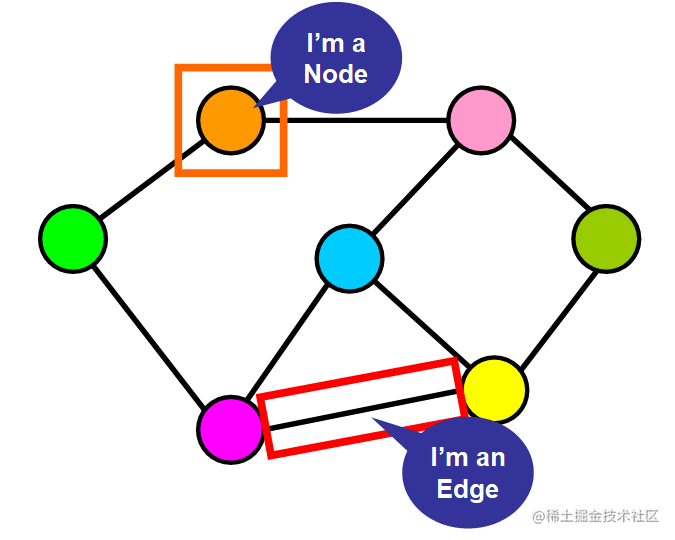#### 2. 符号和术语

• `|V|`=图中顶点（节点）的总数。

• `|E|`=图中的连接总数（边）。

``````|V| = 6
|E| = 7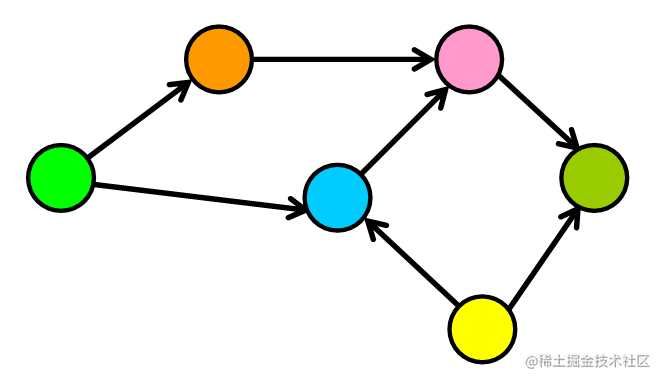#### 3. 有向图与无向图

##### 1. 有向图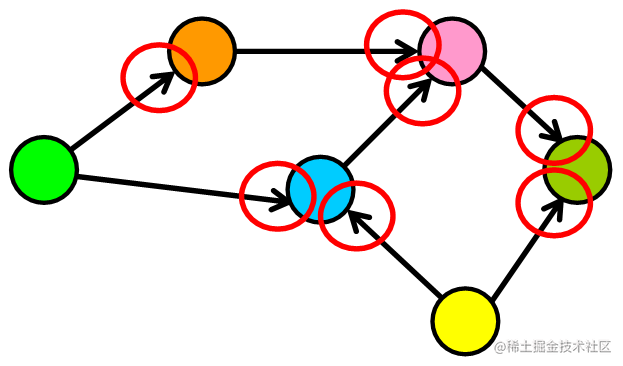##### 2. 无向图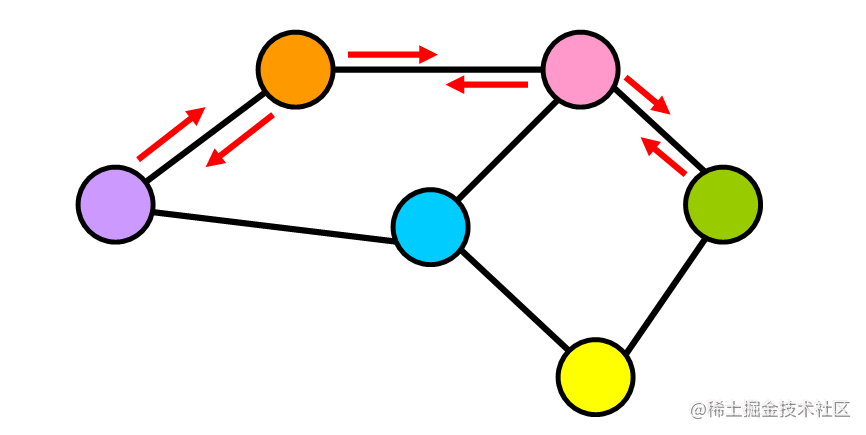#### 4. 加权图

• 权重可以表示距离，时间，社交网络中两个用户之间共享的连接数。
• 或者可以用于描述您正在使用的上下文中的节点之间的连接的任何内容。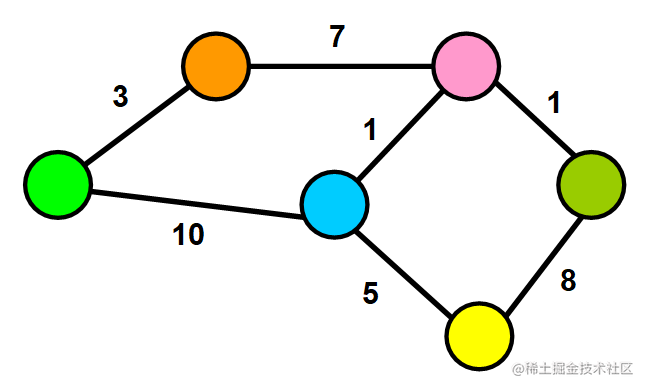#### 5. 稀疏图与密集图#### 6. 循环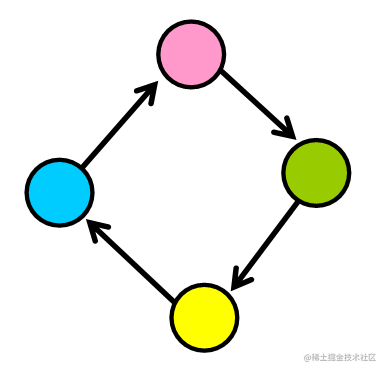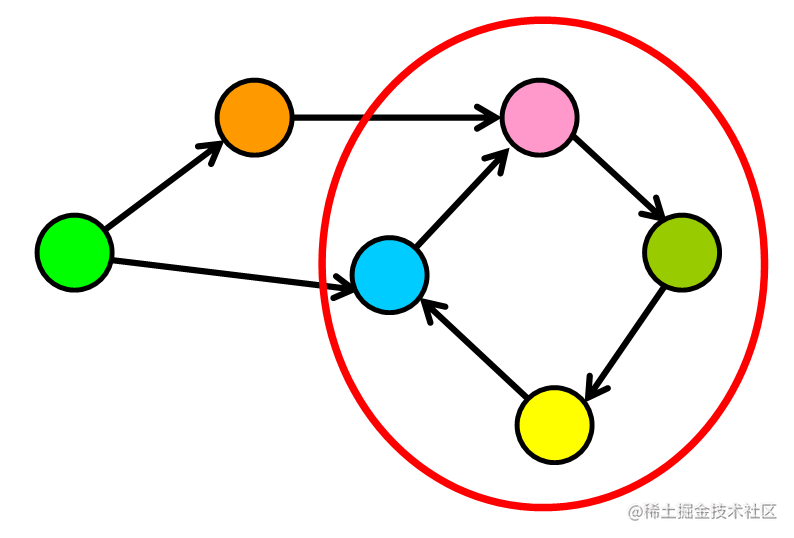### 7.3 图的实现

``````class Graph {
constructor() {
this.AdjList = new Map();
}

// 基础操作方法
// addEdge(vertex, node) {}
// print() {}
}

#### 1. `addVertex`：添加顶点

``````addVertex(vertex) {
} else {
}
}

``````let graph = new Graph();

``````Map {
'A' => [],
'B' => [],
'C' => [],
'D' => []
}

``````Map {
'A' => ['B', 'C', 'D'],
// B没有任何指向
'B' => [],
'C' => ['B'],
'D' => ['C']
}

#### 2. `addEdge`：添加边(`Edge`)

`````` addEdge(vertex, node) {
// 向顶点添加边之前，必须验证该顶点是否存在。
// 确保添加的边尚不存在。
let arr = this.AdjList.get(vertex);
// 如果都通过，那么可以将边添加到顶点。
if(!arr.includes(node)){
arr.push(node);
}
}else {
throw `Can't add non-existing vertex ->'\${node}'`;
}
} else {
throw `You should add '\${vertex}' first`;
}
}

#### 3. `print`：打印图(`Graph`)

``````print() {
for (let [key, value] of this.AdjList) {
console.log(key, value);
}
}

#### 测试一下

``````let g = new Graph();
let arr = ['A', 'B', 'C', 'D', 'E', 'F'];
for (let i = 0; i < arr.length; i++) {
}
g.print();
/* PRINTED */
// A [ 'B', 'D', 'E' ]
// B [ 'C' ]
// C [ 'F' ]
// D [ 'E' ]
// E [ 'F', 'C' ]
// F []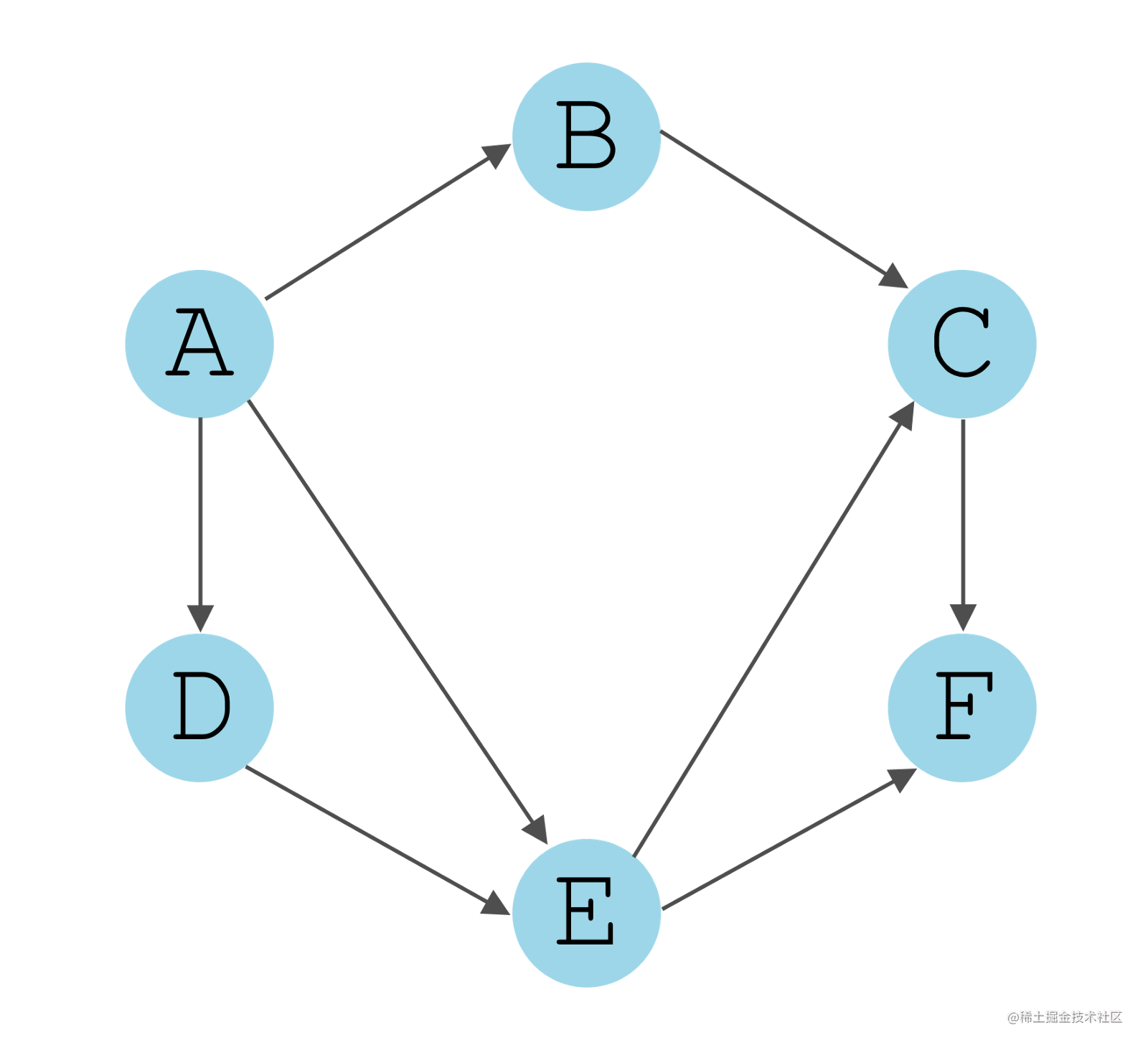• 广度优先算法，`BFS`
• 深度优先算法，`DFS`
• `BFS` 的重点在于队列，而 `DFS` 的重点在于递归。这是它们的本质区别。

#### 5. 广度优先算法实现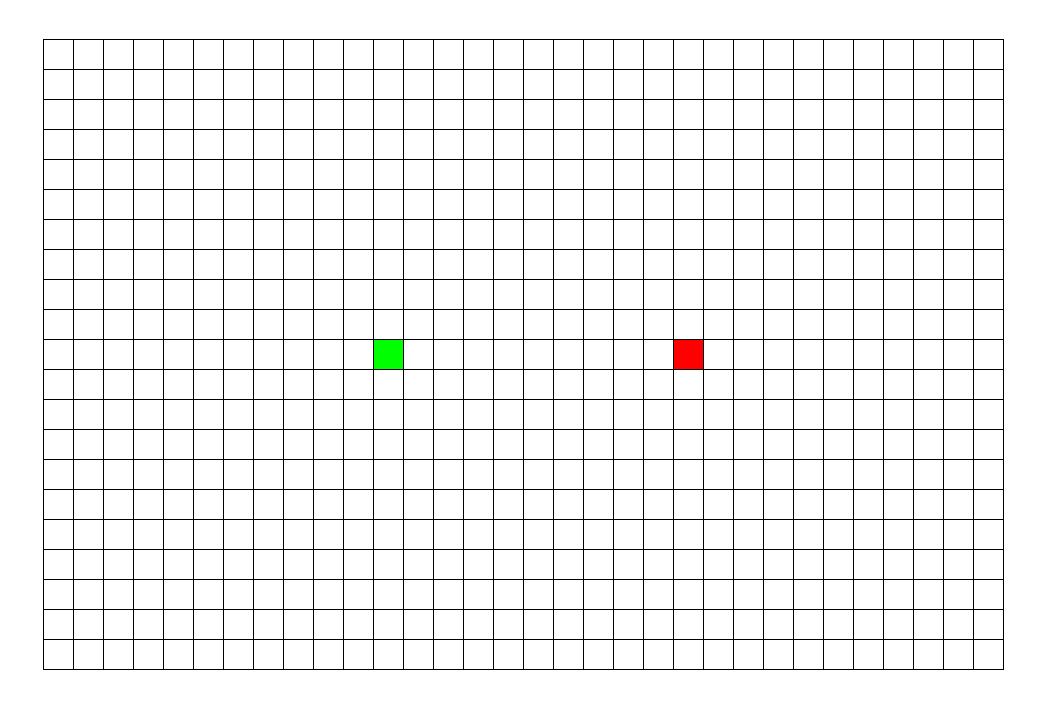如上图所示，从起点出发，对于每次出队列的点，都要遍历其四周的点。所以说 BFS 的搜索过程和 “湖面丢进一块石头激起层层涟漪” 很相似，此即 “广度优先搜索算法” 中“广度”的由来。

• BFS将起始节点作为参数。（例如`'A'`
• 初始化一个空对象：`visited`
• 初始化一个空数组：`q`，该数组将用作队列。
• 将起始节点标记为已访问。`（visited = {'A': true}）`
• 将起始节点放入队列中。`（q = ['A']）`
• 循环直到队列为空

• 从中获取元素`q`并将其存储在变量中。`（let current = q.pop()）`
• 打印 当前 `current`
• 从图中获取`current`的边。`（let arr = this.AdjList.get(current)）`
• 如果未访问元素，则将每个元素标记为已访问并将其放入队列中。
``````visited = {
'A': true,
'B': true,
'D': true,
'E': true
}
q = ['B', 'D', 'E']

``````createVisitedObject(){
let arr = {};
for(let key of this.AdjList.keys()){
arr[key] = false;
}
return arr;
}

bfs(startingNode){
let visited = this.createVisitedObject();
let q = [];

visited[startingNode] = true;
q.push(startingNode);

while(q.length){
let current = q.pop()
console.log(current);

let arr = this.AdjList.get(current);

for(let elem of arr){
if(!visited[elem]){
visited[elem] = true;
q.unshift(elem)
}
}

}
}

#### 6. 深度优先算法实现

• 接受起点作为参数`dfs(startingNode)`
• 创建访问对象`let visited = this.createVisitedObject() `
• 调用辅助函数递归起始节点和访问对象`this.dfsHelper(startingNode, visited) `
• `dfsHelper` 将其标记为已访问并打印出来。
``````createVisitedObject(){
let arr = {};
for(let key of this.AdjList.keys()){
arr[key] = false;
}
return arr;
}

dfs(startingNode){
console.log('\nDFS')
let visited = this.createVisitedObject();
this.dfsHelper(startingNode, visited);
}

dfsHelper(startingNode, visited){
visited[startingNode] = true;
console.log(startingNode);

let arr = this.AdjList.get(startingNode);

for(let elem of arr){
if(!visited[elem]){
this.dfsHelper(elem, visited);
}
}
}

doesPathExist(firstNode, secondNode){
let path = [];
let visited = this.createVisitedObject();
let q = [];
visited[firstNode] = true;
q.push(firstNode);
while(q.length){
let node = q.pop();
path.push(node);
let elements = this.AdjList.get(node);
if(elements.includes(secondNode)){
console.log(path.join('->'))
return true;
}else{
for(let elem of elements){
if(!visited[elem]){
visited[elem] = true;
q.unshift(elem);
}
}
}
}
return false;
}
}

`Vans`，下一个。

## 8. 字典树：`Trie`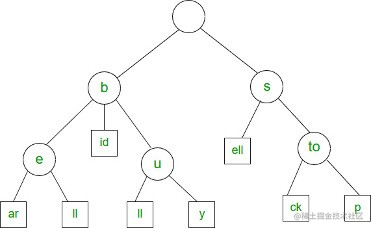`Trie`（通常发音为“try”）是针对特定类型的搜索而优化的树数据结构。当你想要获取部分值并返回一组可能的完整值时，可以使用`Trie`。典型的例子是自动完成。`Trie`，是一种搜索树，也称字典树或单词查找树，此外也称前缀树，因为某节点的后代存在共同的前缀。

• key都为字符串，能做到高效查询和插入，时间复杂度为`O(k)`，k为字符串长度
• 缺点是如果大量字符串没有共同前缀时很耗内存。
• 它的核心思想就是减少没必要的字符比较，使查询高效率。
• 即用空间换时间，再利用共同前缀来提高查询效率。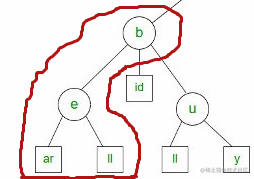• 自动填充/预先输入
• 搜索
• 输入法选项
• 分类

• IP地址检索
• 电话号码
• 以及更多...

### 8.2 字典树的实现

``````class PrefixTreeNode {
constructor(value) {
this.children = {};
this.endWord = null;
this.value = value;
}
}
class PrefixTree extends PrefixTreeNode {
constructor() {
super(null);
}
// 基础操作方法
// predictWord(string) {}
// logAllWords() {}
}

#### 1. `addWord`: 创建一个节点

``````addWord(string) {
const addWordHelper = (node, str) => {
if (!node.children[str]) {
node.children[str] = new PrefixTreeNode(str);
if (str.length === 1) {
node.children[str].endWord = 1;
} else if (str.length > 1) {
}
};
}

#### 2. `predictWord`：预测单词

``````predictWord(string) {
let getRemainingTree = function(string, tree) {
let node = tree;
while (string) {
node = node.children[string];
string = string.substr(1);
}
return node;
};

let allWords = [];

let allWordsHelper = function(stringSoFar, tree) {
for (let k in tree.children) {
const child = tree.children[k]
let newString = stringSoFar + child.value;
if (child.endWord) {
allWords.push(newString);
}
allWordsHelper(newString, child);
}
};

let remainingTree = getRemainingTree(string, this);
if (remainingTree) {
allWordsHelper(string, remainingTree);
}

return allWords;
}

#### 3. `logAllWords`：打印所有的节点

``````  logAllWords() {
console.log('------ 所有在字典树中的节点 -----------')
console.log(this.predictWord(''));
}

`logAllWords`，通过在空字符串上调用`predictWord`来打印`Trie`中的所有节点。

## 9. 散列表（哈希表）：`Hash Tables`

### 9.2 哈希表的构成

`Hash Tables`优化了键值对的存储。在最佳情况下，哈希表的插入，检索和删除是恒定时间。哈希表用于存储大量快速访问的信息，如密码。

``````{[[['a'，9]，['b'，88]]，[['e'，7]，['q'，8]]，[['j'，7]，['l '，8]]]};

• 外部数组有多个等于数组最大长度的桶（子数组）。
• 在桶内，元组或两个元素数组保持键值对。

### 9.3 哈希表的基础知识

• 在大学中，每个学生都会被分配一个唯一的卷号，可用于检索有关它们的信息。
• 在图书馆中，每本书都被分配了一个唯一的编号，可用于确定有关图书的信息，例如图书馆中的确切位置或已发给图书的用户等。

#### 2, 一个哈希表的诞生

1. 在散列中，通过使用散列函数将大键转换为小键。
2. 然后将这些值存储在称为哈希表的数据结构中。
3. 散列的想法是在数组中统一分配条目（键/值对）。为每个元素分配一个键（转换键）。
4. 通过使用该键，您可以在`O(1)`时间内访问该元素。
5. 使用密钥，算法（散列函数）计算一个索引，可以找到或插入条目的位置。

• 通过使用散列函数将元素转换为整数。此元素可用作存储原始元素的索引，该元素属于哈希表。
• 该元素存储在哈希表中，可以使用散列键快速检索它。
``````hash = hashfunc（key）
index = hash ％ array_size

#### 3. 哈希函数

• 哈希函数是可用于将任意大小的数据集映射到固定大小的数据集的任何函数，该数据集属于散列表
• 哈希函数返回的值称为哈希值，哈希码，哈希值或简单哈希值。

1. 易于计算：它应该易于计算，并且不能成为算法本身。
2. 统一分布：它应该在哈希表中提供统一分布，不应导致群集。
3. 较少的冲突：当元素对映射到相同的哈希值时发生冲突。应该避免这些。

#### 4. 良好的哈希函数

• `a，b，c，d，e``f``ASCII`值分别为`97,98,99,100,101``102`，总和为：`597`
• `597`不是素数，取其附近的素数`599`，来减少索引不同字符串（冲突）的可能性。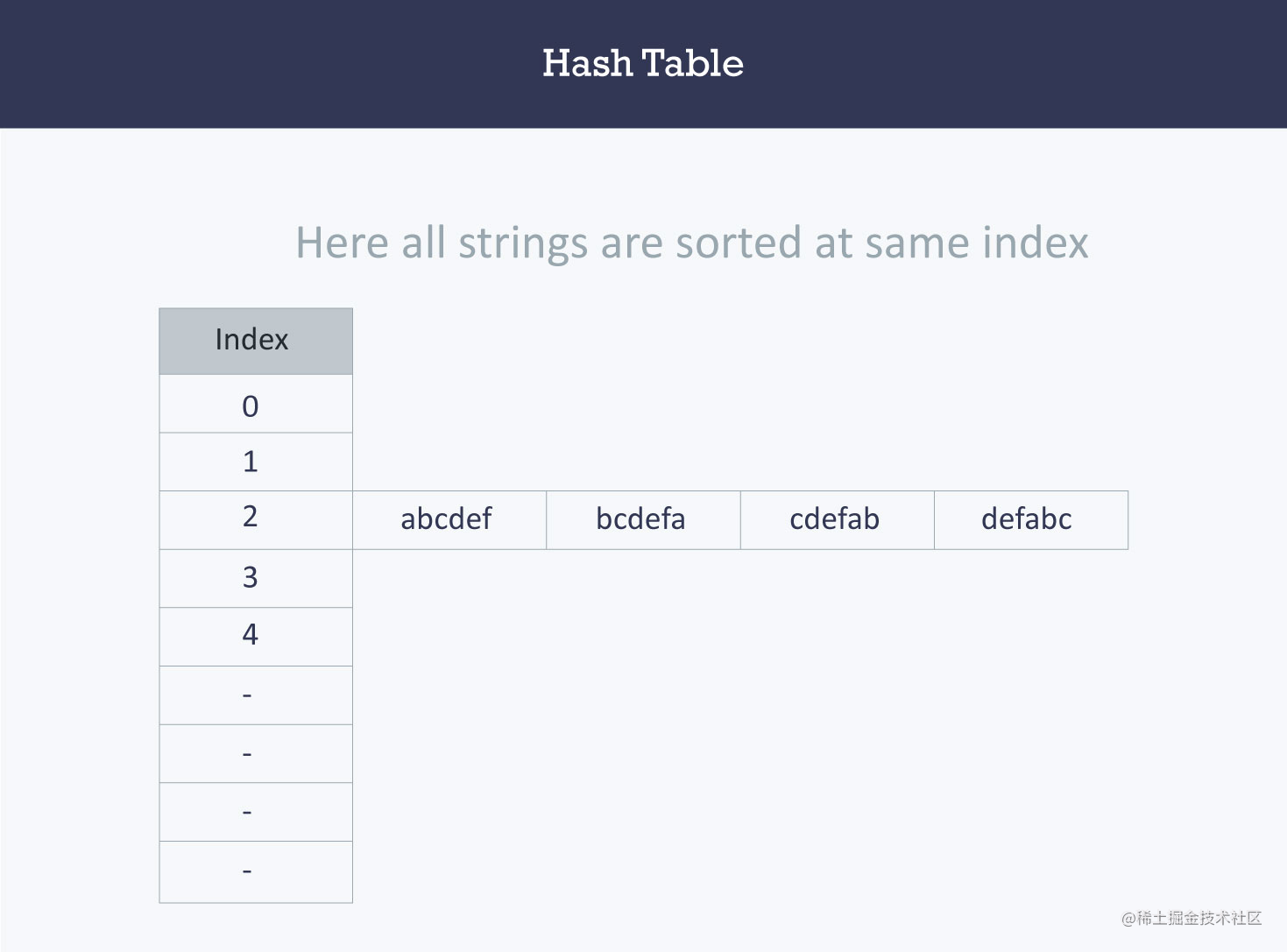• 这里，访问特定字符串需要`O(n)`时间（其中n是字符串数）。
• 这表明该哈希函数不是一个好的哈希函数。

``````{“abcdef”，“bcdefa”，“cdefab”，“defabc”}

• 都是由`a，b，c，d，e``f`组成
• 不同点在于组成顺序。

• 特定字符串的索引将等于字符的ASCII值之和乘以字符串中它们各自的顺序
• 之后将它与`2069`（素数）取余。

abcdef（97 1 + 98 2 + 99 3 + 100 4 + 101 5 + 102 6）％206938
bcdefa（98 1 + 99 2 + 100 3 + 101 4 + 102 5 + 97 6）%206923
cdefab（99 1 + 100 2 + 101 3 + 102 4 + 97 5 + 98 6）％206914
defabc（100 1 + 101 2 + 102 3 + 97 4 + 98 5 + 99 6）%206911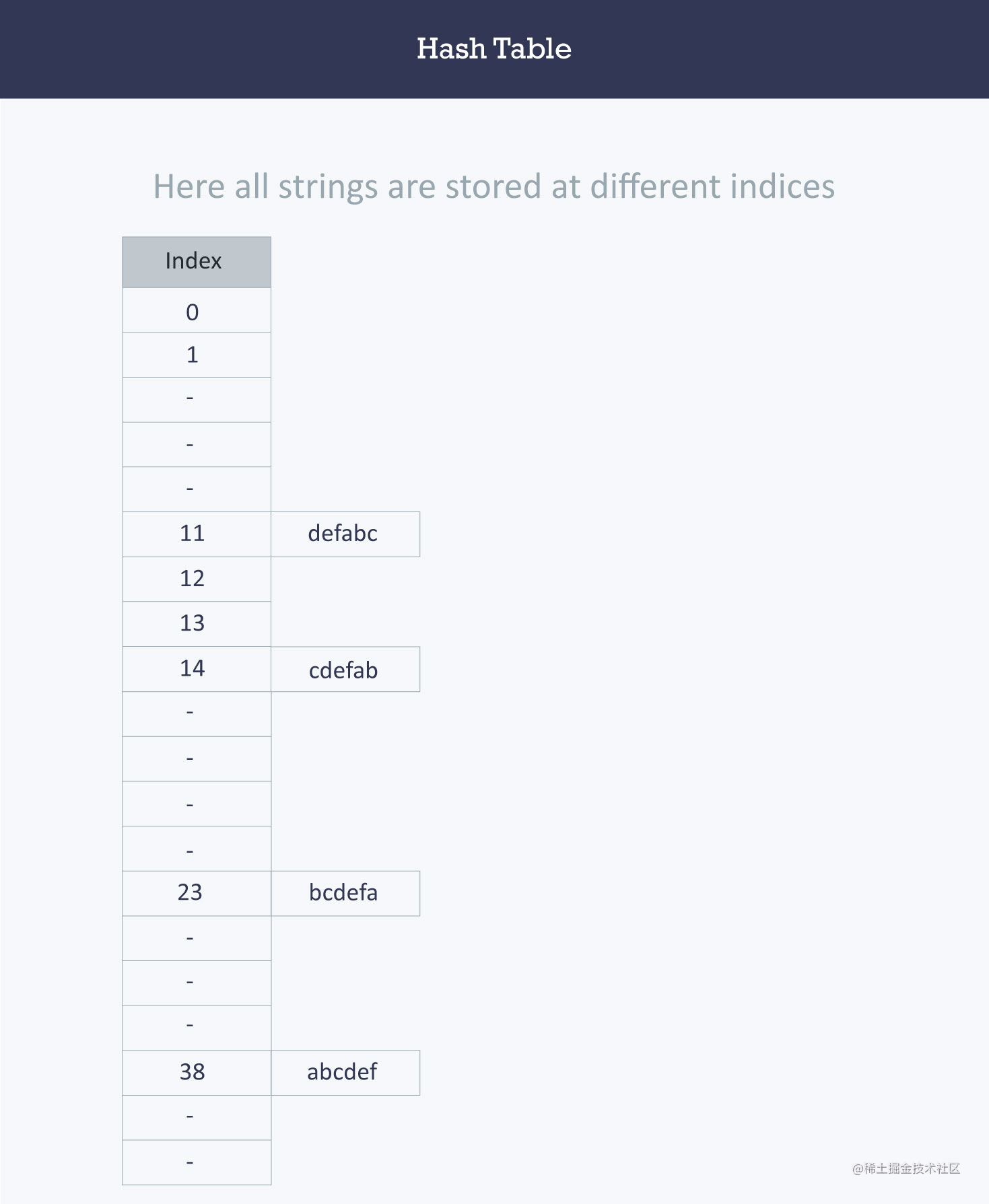### 9.4 哈希表的实现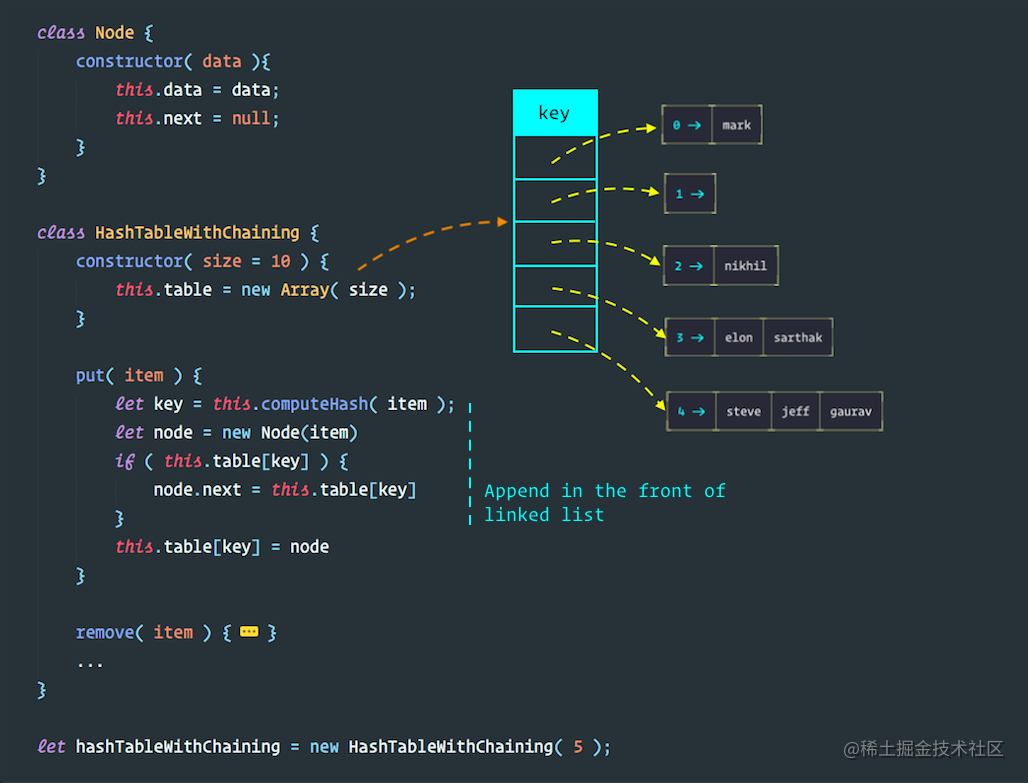``````class Node {
constructor( data ){
this.data = data;
this.next = null;
}
}

class HashTableWithChaining {
constructor( size = 10 ) {
this.table = new Array( size );
}

// 操作方法
// computeHash( string ) {...}
// ...
}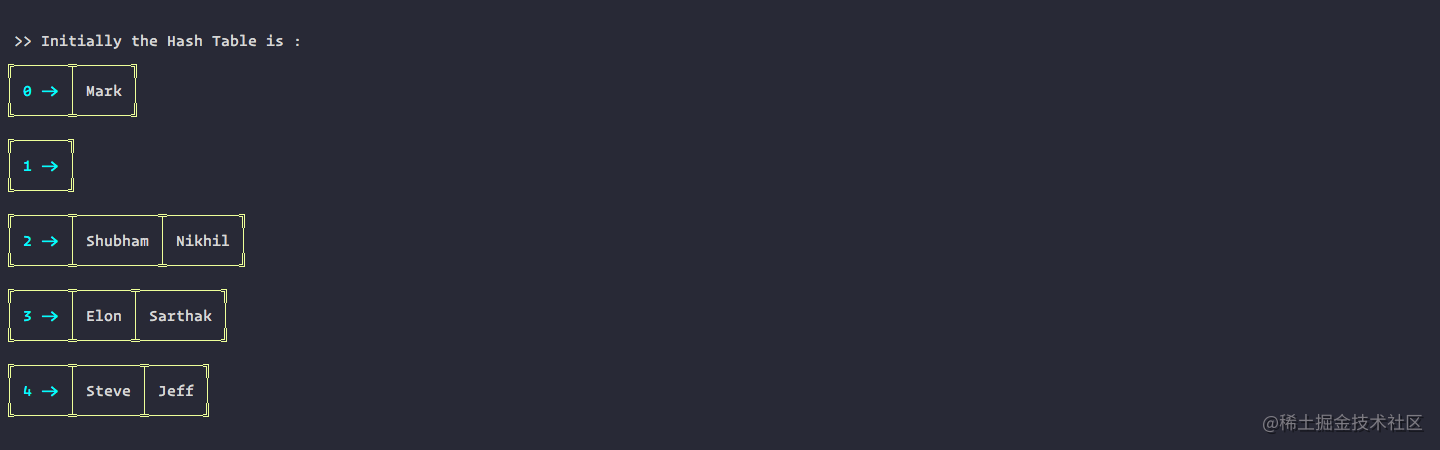#### 1. `isPrime`：素数判断

``````isPrime( num ) {
for(let i = 2, s = Math.sqrt(num); i <= s; i++)
if(num % i === 0) return false;
return num !== 1;
}

#### 2. `computeHash|findPrime`：哈希函数生成

``````computeHash( string ) {
let H = this.findPrime( this.table.length );
let total = 0;
for (let i = 0; i < string.length; ++i) {
total += H * total + string.charCodeAt(i);
}
}
// 取模
findPrime( num ) {
while(true) {
if( this.isPrime(num) ){ break; }
num += 1
}
return num;
}

#### 3. `Put`：插入值

``````put( item ) {
let key = this.computeHash( item );
let node = new Node(item)
if ( this.table[key] ) {
node.next = this.table[key]
}
this.table[key] = node
}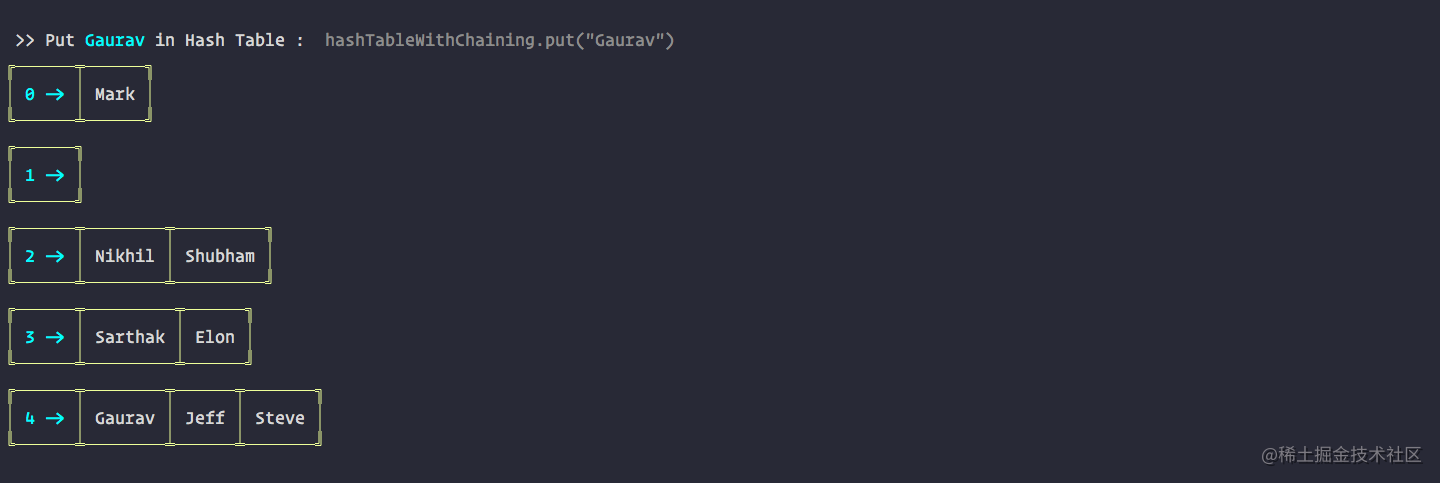#### 4. `Remove`：删除值

``````remove( item ) {
let key = this.computeHash( item );
if( this.table[key] ) {
if( this.table[key].data === item ) {
this.table[key] = this.table[key].next
} else {
let current = this.table[key].next;
let prev = this.table[key];
while( current ) {
if( current.data === item ) {
prev.next = current.next
}
prev = current
current = current.next;
}
}
}
}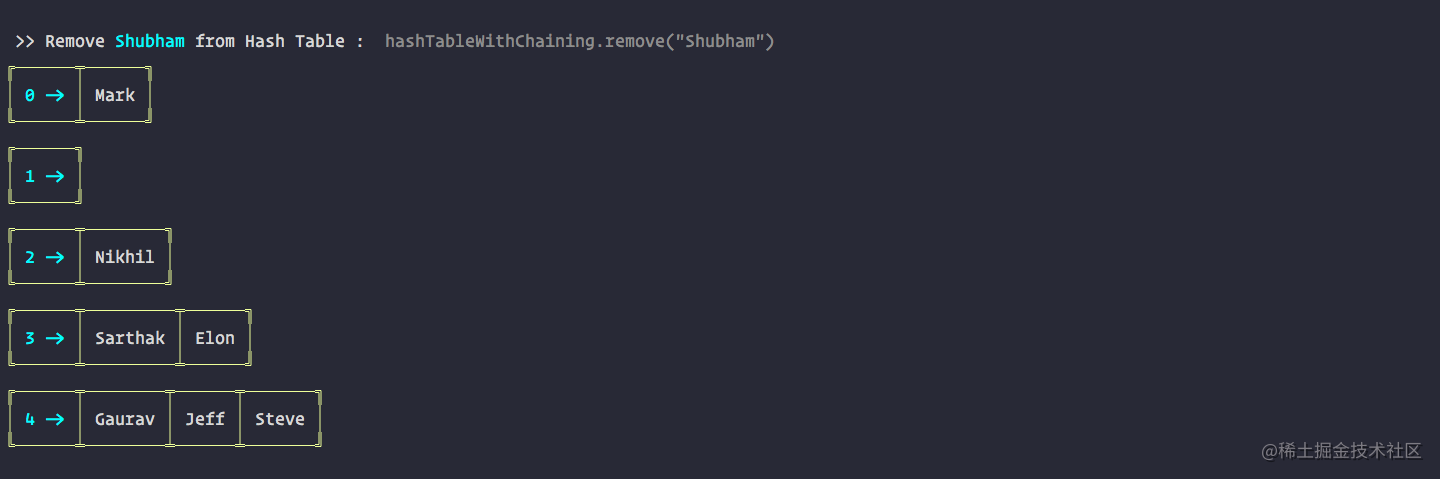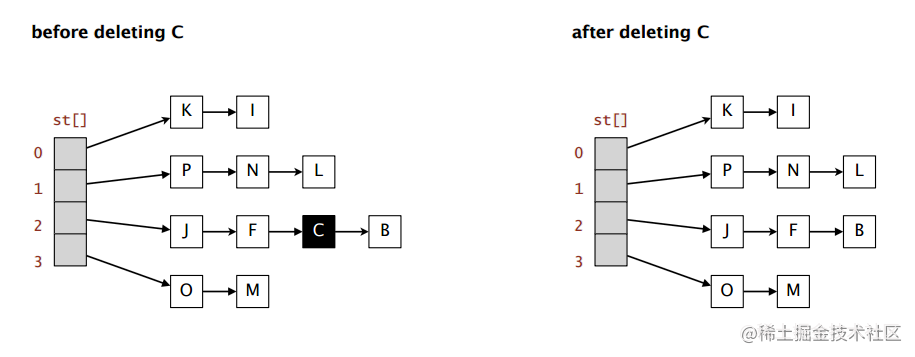#### 5. `contains`：判断包含

``````contains(item) {
for (let i = 0; i < this.table.length; i++) {
if (this.table[i]) {
let current = this.table[i];
while (current) {
if (current.data === item) {
return true;
}
current = current.next;
}
}
}
return false;
}

#### 6. `Size & IsEmpty`：判断长度或空

``````size( item ) {
let counter = 0
for(let i=0; i<this.table.length; i++){
if( this.table[i] ) {
let current = this.table[i]
while( current ) {
counter++
current = current.next
}
}
}
return counter
}
isEmpty() {
return this.size() < 1
}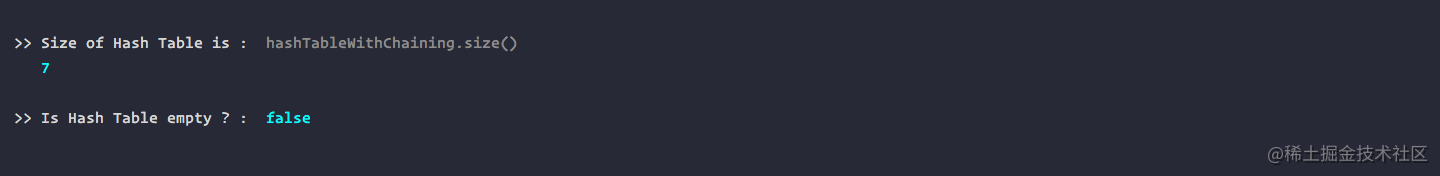#### 7. `Traverse`：遍历

``````traverse( fn ) {
for(let i=0; i<this.table.length; i++){
if( this.table[i] ) {
let current = this.table[i];
while( current ) {
fn( current );
current = current.next;
}
}
}
}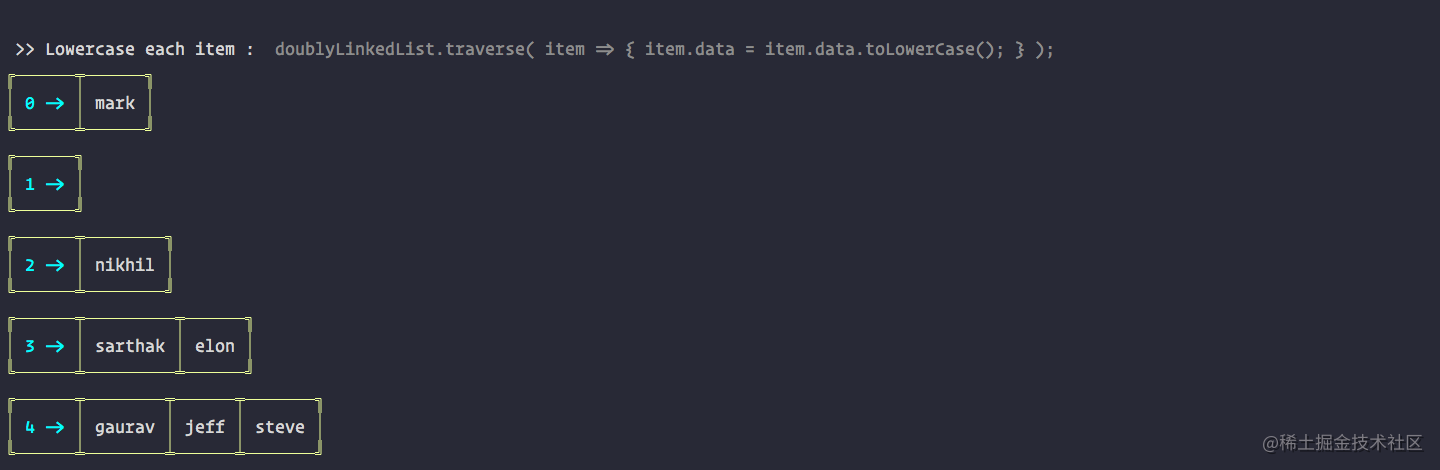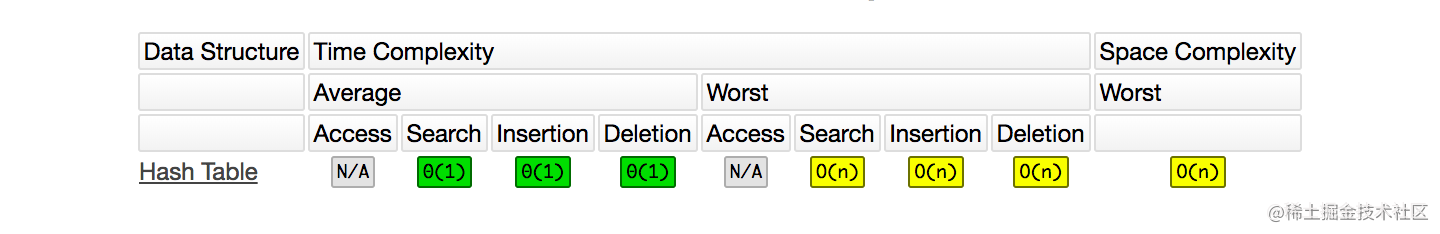## 求一份深圳的内推目前本人在(又)准备跳槽，希望各位大佬和HR小姐姐可以内推一份靠谱的深圳前端岗位！

• 微信：`huab119`
• 邮箱：`454274033@qq.com`

## ❤️ 看完三件事

1. 点赞，让更多的人也能看到这篇内容（收藏不点赞，都是耍流氓 -_-）
2. 关注公众号「前端劝退师」，不定期分享原创知识。
3. 也看看其它文章